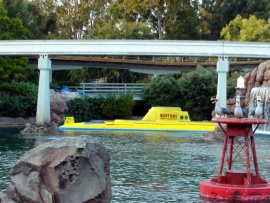This Disney wallpaper is based on the Disneyland ride, Finding Nemo Submarine Voage. Taken in May, 2010, you can see one of the submarines about to into the cave. Above the lake you can see the monorail track and in behind the submarine you can see a blue Autopia cars going across the bridge. This Disneyland ride is based on the 2003 Pixar and Disney movie, Finding Nemo. Image copyright: FreeComputerWallpapers.net Do not use on another site without permission.### function roundNumber(b,c){var a=Math.round(b*Math.pow(10,c))/Math.pow(10,c);return a}function resPrints(){var b=screen.width;var c=screen.height;var a=roundNumber(b/c,2);var d="Your display Resolution: <strong> "+b+" x "+c+" </strong> | Aspect Ratio:<strong> ";if(a==1.25||a==1.33){d+="Standard 4:3"}else{if(a==1.6||a==1.67){d+="Wide 8:5"}else{if(a==1.78){d+="HD 16:9"}else{if(a==1.76){d+="Mobile PSP"}else{if(a==0.75||(a==1.33&&b<=640)){d+="Mobile VGA"}else{if(a==0.6||(a==1.67&&b<=800)){d+="Mobile WVGA"}else{if(a==0.67||(a==1.5&&b==480)){d+="Mobile iPhone"}else{if(a==0.8){d+="Mobile Phone"}else{d="Unknown"}}}}}}}}d+="</strong>";document.write(d)}; resPrints();

Wallpaper details:
Added: Wednesday, 04 May 2011 23:39